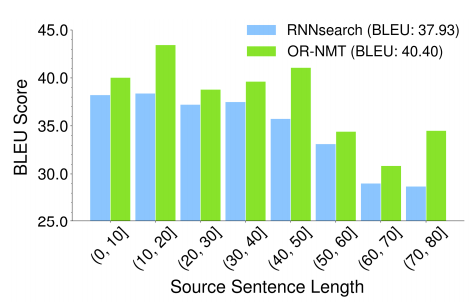# Introduction

• During Training, ground truth words as context. At inference, self-generated words as context.

This discrepancy, called exposure bias, leads to a gap between training and inference. As the target sequence grows, the errors accumulate among the sequence and the model has to predict under the condition it has never met at training time.

• Over-correction, if we only use self-generated words as context.
• A sentence usually has multiple reasonable translations and it cannot be said that the model makes a mistake even if it generates a word different from the ground truth word. For example:

reference: We should comply with the rule.

cand1: We should abide with the rule.
cand2: We should abide by the law.
cand3: We should abide by the rule.

# Problem

At training time, Neural Machine Translation predicts with the ground truth words as context while at inference it has to generate the entire sequence from scratch. This discrepancy of the fed context leads to error accumulation among the way. Furthermore, word-level training requires strict matching between the generated sequence and the ground truth sequence which leads to overcorrection over different but reasonable translations.

# How it Solves

The main framework is to feed as context either the ground truth words or the previous predicted words, i.e. oracle words, with a certain probability. This potentially can reduce the gap between training and inference by training the model to handle the situation which will appear during test time.

## Approach

Oracle Translation Generation

Select an oracle word $y_{j−1}^{oracle}$ at word level or sentence level at the $\{j−1\}^{-th}$ step.

1. Word Level Oracle (SO)

Generally, at the $j$-th step, the NMT model needs the ground truth word $y_{j−1}^*$ as the context word to predict $y_j$ , thus, we could select an oracle word $y_{j−1}^{oracle}$ to simulate the context word. The oracle word should be a word similar to the ground truth or a synonym. Using different strategies will produce a different oracle word $y_{j−1}^{oracle}$. One option is that word-level greedy search could be employed to output the oracle word of each step, which is called Word-level Oracle (called WO).

Gumbel noise, treated as a form of regularization, is added to $o_{j−1}$.

\begin{align*} \eta&= -\log (-\log u) \\ \tilde{o}_{j-1}&= \left(o_{j-1}+\eta\right) / \tau \\ \tilde{P}_{j-1}&= \operatorname{softmax}\left(\tilde{o}_{j-1}\right) \end{align*}

where $\eta$ is the Gumbel noise calculated from a uniform random variable ${u} ∼ {U(0, 1)}$; $\tau$ is temperature. As $\tau$ approaches 0, the ${softmax}$ function is similar to the ${argmax}$ operation, and it becomes uniform distribution gradually when $\tau$ $\rightarrow$ $\infty$.

2. Sentence Level Oracle (SO)

• Generate $top(k)$ translation by beam search.
• Re-rank $top(k)$ translation with BLEU.
• Select $top(i)$.

As the model samples from ground truth word and the sentence-level oracle word at each step, the two sequences should have the same number of words. Force decoding is used to make sure the two sequences have the same length.

Context Sampling with Decay

Employ a sampling mechanism to randomly select the ground truth word $y_{j-1}^*$ or the oracle word $y_{j−1}^{oracle}$ as $y_{j−1}$. At the beginning of training, as the model is not well trained, using $y_{j−1}^{oracle}$ as $y_{j−1}$ too often would lead to very slow convergence, even being trapped into local optimum.

On the other hand, at the end of training, if the context $y_{j−1}$ is still selected from the ground truth word $y_{j-1}^{*}$ at a large probability, the model is not fully exposed to the circumstance which it has to confront at inference and hence can not know how to act in the situation at inference. In this sense, the probability $p$ of selecting from the ground truth word can not be fixed, but has to decrease progressively as the training advances. At the beginning, $p=1$, which means the model is trained entirely based on the ground truth words. As the model converges gradually, the model selects from the oracle words more often.

where \begin{align*}{p} = \frac{\mu}{\mu + \exp(\mathcal{e}/\mu)}\end{align*}

Here, $\mathcal{e}$ corresponds to the epoch number and $\mu$ is a hyper-parameter. The function is strictly monotone decreasing. As the training proceeds, the probability $p$ of feeding ground truth words decreases gradually.

## Training

The objective is to maximize the probability of the ground truth sequence based on maximum likelihood estimation (MLE). Thus, following loss function is minimized:

\begin{align*}\mathcal{L}(\theta)=-\sum_{n=1}^{N} \sum_{j=1}^{\left|\mathbf{y}^{n}\right|} \log P_{j}^{n}\left[y_{j}^{n}\right]\end{align*}

where ${N}$ is the number of sentence pairs in the training data, $|y^n|$ indicates the length of the ${n}$-th ground truth sentence, $P_j^n$ refers to the predicted probability distribution at the ${j}$-th step for the ${n}$-th sentence, hence $P_j^n[y_j^n]$ is the probability of generating the ground truth word $y_j^n$ at the ${j}$-th step.

# Results

Based on the RNNSearch, the authors introduced the word-level oracles, sentence-level oracles and the Gumbel noises to enhance the overcorrection recovery capacity. They split the translations for the MT03 test set into different bins according to the length of source sentences, then test the BLEU scores for translations in each bin separately. Their approach can achieve big improvements over the baseline system in all bins, especially in the bins (10,20], (40,50] and (70,80] of the super-long sentences.Performance comparison on the MT03 test set with respect to the different lengths of source sentences on the Zh→En translation task.

# Future Scope

Reporting of NIST or ROUGE scores would be helpful for comparison purposes as BLEU doesn’t consider sentence structure.

# Takeaways

• Sampling as context from the ground truth and the generated oracle can mitigate exposure bias.
• Sentence-level oracle is better than word-level oracle.
• Gumbel noise can help improve translation quality.
1. Zhang, W., Feng, Y., Meng, F., You, D., & Liu, Q. (2019). Bridging the Gap between Training and Inference for Neural Machine Translation. Proceedings of the 57th Annual Meeting of the Association for Computational Linguistics, 4334–4343. https://doi.org/10.18653/v1/P19-1426